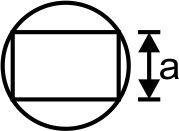#### From a solid sphere of mass M and radius R a cube of maximum possible volume is cut. Moment of inertia of cube about an axis passing through its center and  perpendicular to one of its faces is : Option 1)Option 2)Option 3)Option 4)As we learnt in

Moment of inertia for system of particle -- wherein

Applied when masses are placed discretely.M.O.I. of the cube about the given axis.Option 1)This is an incorrect option.

Option 2)This is an incorrect option.

Option 3)This is the correct option.

Option 4)This is the correct option.

#### perimeter